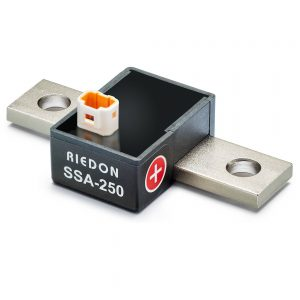Select PageResistors are one of the most basic and essential electronic components. They control the current flow in a circuit and limit the amount of current that can flow through a particular component. A current sense resistor is a type of resistor specifically designed to measure the current flowing through it.

What Are Current Sense Resistors?

Current sense resistors are electronic components that are used to measure current in an electrical circuit. They are usually made of thin metal blades or metal film and placed in the path of the current to be measured. The Voltage output of the current sense resistor changes with the current, and this change can be measured to determine the current flowing through the circuit.

Current sense resistors are used in various applications, including power supplies, motor controllers, and overcurrent protection circuits. They are often used in conjunction with op-amps or other amplifiers to increase the strength of the measurement. Current sense resistors are available in various sizes and shapes, and their resistance values can be customized to meet the application’s needs.

How Do Current Sense Resistors Work?

The current sense resistor is placed in series with the circuit that is being measured. The voltage across the resistor is then measured. This voltage is proportional to the current flowing through the resistor. By knowing the resistance value of the current sense resistor, the current flowing through the circuit can be calculated.

How Do You Choose the Right Current Sense Resistors?

Choosing the correct current resistor is critical to any current sensing application. There are many factors to consider, including resistance value, power rating, temperature coefficient, and tolerance.

1. Resistance Value

This is the most important factor to consider when choosing a current sense resistor. The resistance value determines the amount of voltage drop across the resistor, which is proportional to the current flowing through it. For example, a 0.1Ω resistor will produce a 100mV voltage drop for every 1 Amp of current flowing through it.

1. Power Rating

The power rating of a resistor is the maximum amount of power it can dissipate without being damaged. It is essential to choose a resistor with a power rating that is high enough to handle the maximum amount of current that will flow through it.

1. Temperature Coefficient

The temperature coefficient of a resistor is the amount that its resistance value will change for every degree change in temperature. It is important to choose a resistor with a low-temperature coefficient to minimize the effects of temperature changes on the voltage drop across the resistor.

1. Tolerance

Tolerance is the amount a resistor’s resistance value can vary from its nominal value. Choosing a resistor with a low tolerance is vital to ensure that the voltage drop across the resistor is as accurate as possible.

Final Thoughts

Current sense resistors are an essential component in many electrical and electronic devices. They help improve efficiency by allowing devices to measure and control current flow accurately. By understanding how current sense resistors work, we can better appreciate their role in keeping our devices running smoothly and efficiently.

If you need current sense resistors, you should check out Riedon, a reliable resistor manufacturer. Go through the wide range of available products on our website and place your order today!